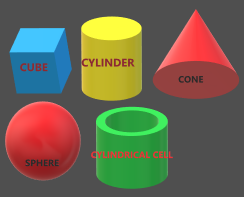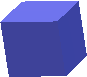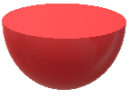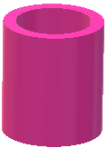### Resource Centre Mathematics

Resource Centre Mathematics Mathematics worksheet, mathematics basic points and formulas, mathematics lesson plan, mathematics multiple choice questions Workplace Dashboard CBSE Syllabus For Session 2023-24 For  :   Classes IX & X    |   Classes XI & XII Watch Videos on Maths Solutions CLASS IX MATHEMATICS FORMULAS &  BASIC CONCEPTS

# Chapter 13 For Class IX & X

### Surface Area and volume of Cube, Cuboid, Cylinder, Cone, Sphere, Hemi-Sphere, Frustum of Cone, Cylindrical Pipe, useful formulas for surface area and volume of solid figuresEveryday we have seen different materials and shapes in our surroundings. All that materials which we can touch and make use in our daily work are called solid figures.

These are also be called solid shapes because all these figures are involve three dimensions.

Further these are classified into different types. Main types are cube, cuboid, cylinder, cone, sphere, hemi-sphere and frustum of cone.

Here in this topic we discussed surface area and volume of these shapes.

Note:
• Students of  IX standard learn all the formulas except Frustum of Cone.
• Frustum of cone is only for the students of X standard.

 SOLID FIGURE CUBECurved Surface area of cube = 4(side)2 Total surface area of cube = (side)2 Volume of Cube = (side)3  Diagonal of Cube = √3(side)2Number of faces = 6Number of vertices = 8 Number of Edges = 12 Each face of a cube is a square CUBOIDCurved Surface Area of cuboid = 2(l + b) ✖ hTotal Surface Area of cuboid = 2(lb + bh + hl)Volume of cuboid = Length ✖ Breadth ✖ HeightDiagonal of  cuboid =Number of faces = 6  Number of vertices = 8Number of Edges = 12 Each face of a cube is a rectangle CYLINDERCurved Surface Area of cylinder = 2πrhTotal Surface Area of cylinder = 2πr(r + h)Volume of cylinder = πr2hNumber of faces = 3 (One curved surface and two plane surface)Number of vertices = 0 Number of edges = 2 CONECurved Surface Area = πrl Total Surface Area = πr(r + l) Volume =Number of faces = 2 (One curved surface and one plane surface)Number of vertices = 1Number of edges = 1 SPHERECurved Surface Area = 4πr2Total Surface Area =  4πr2 Volume =Number of faces = 1 (One curved surface ) Number of vertices = 0Number of edges = 0 HEMI-SPHERECurved Surface Area = 2πr2 Total Surface Area = 3πr2Volume =Number of faces = 2 (One curved surface and one plane surface)Number of vertices = 0Number of edges = 1 HOLLOW  CYLINDRICAL PIPE     Inner Curved Surface Area = 2πrh Outer Curved Surface Area = 2πRh Area of two Rims = 2πR2 - 2πr2 Total Surface Area = 2πrh + 2πRh + (2πR2 - 2πr2)Volume = πh(R2 - 2πr2) Number of faces = 4 (Inner and outer curved surface, top and bottom plane surface) Number of vertices = 0 Number of edges = 4 FRUSTUM OF  CONE Note: This topic is only for the students of X standardCurved Surface Area = πl(r1 + r2)Where,  Total Surface Area = πl(r1 + r2) +   +  Volume =Number of faces = 3 (One curved surface and two plane surface)Number of vertices = 0Number of edges = 2

Surface area and volumes

 Solid Figure Curved Surface Area Total Surface Area Volume CUBE $4\times \left ( side \right )^{2}$ $6\times \left ( side \right )^{2}$ $\left ( side \right )^{3}$ CUBOID $2\left ( l+b \right )\times h$ $2\left ( lb+bh+hl \right )$ $lbh$ CYLINDE $2\pi rh$ $2\pi rh+\pi r^{2}+\pi r^{2}$$=2\pi r\left ( r+h \right )$ $\pi r^{2}h$ CONE $\pi rl$ $\pi r\left ( r+l \right )$ $\frac{1}{3}\pi r^{2}h$ SPHERE $4\pi r^{2}$ $4\pi r^{2}$ $\frac{4}{3}\pi r^{3}$ HEMI - SPHERE $2\pi r^{2}$ $3\pi r^{2}$ $\frac{2}{3}\pi r^{3}$ FRUSTUM OF CONE $\pi l\left ( r_{1}+r_{2} \right )$ $\pi l\left ( r_{1}+r_{2} \right )+\pi r_{1}^{2}+\pi r_{2}^{2}$ $\frac{1}{3}\pi h\left [ r_{1}^{2}+r_{2}^{2}+r_{1}r_{2} \right ]$

👉While discussing surface area and volume unit conversion is an essential part. Without the knowledge of units students can not achieve the objective of the topic and they may face difficulty and mistakes most of the time. So in order to understand the techniques of unit conversion students click here

1.2.